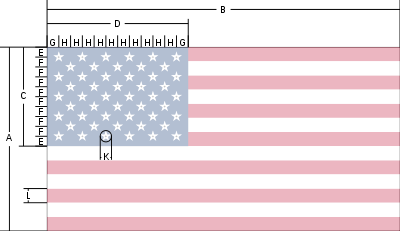# How to Draw the USA Flag

13 NovThis is one a series of posts on how to draw world flags. Finding the specifications for these flags, and attempting to reproduce the flag perfectly, according to the specifications, is a great way to teach yourself basic graphics programming.

Previously: Australia

Having figured out how to draw stars, and having drawn plenty of stripes, we are ready to tackle the Stars and Stripes. The specification on Wikipedia provides a handy chart and some useful measurements, which I’ve reworded a bit for consistency (I always use ‘width’ for horizontal measurements and height for ‘vertical’ measurements).• Hoist (height) of the flag: A = 1.0
• Fly (width) of the flag: B = 1.9
• Hoist (height) of the Union: C = 0.5385 (A × 7/13, spanning seven stripes)
• Fly (width) of the Union: D = 0.76 (B × 2/5, two fifths of the flag width)
• E = F = 0.0538 (C/10, One tenth of the height of the Union)
• G = H = 0.0633 (D/12, One twelfth of the width of the Union)
• Diameter of star: K = 0.0616
• Height of stripe: L = 0.0769 (A/13, One thirteenth of the flag height)

I’m going to base all my numbers on the height (or hoist), which you can change at the top of the script.

```int kHeight = 260;
```

My colors come from the wikipedia specification.

```color blueColor  = #3C3B6E;
color whiteColor = #FFFFFF;
color redColor   = #B22234;
```

When setting the size of the sketch, I use the specified 1.9:1 aspect ratio.

```  size(round(kHeight*1.9), kHeight);
```

In the draw function, we set some useful measurements, that come from the specification.

```  int  nbrStripes = 13;
float stripeHeight = height/(float) nbrStripes;
float unionWidth = width * 2/5.0;
float unionHeight= height * 7/13.0;
```

We first fill the frame with white, and then draw our 13 stripes.

```  background(whiteColor);

fill(redColor);
for (int i = 0; i < nbrStripes; i += 2) {
rect(0,stripeHeight*i, width, stripeHeight);
}
```

This produces the following image.

Notice that I could have drawn the top stripes short, but it needlessly complicates the code. It's simpler to just draw them all the way across, and then draw the blue union on top of them, as I do in the next step.

```  fill(blueColor);
rect(0,0,unionWidth, unionHeight);
```

Finally, we draw the stars. This is the only complicated bit, as the stars are interspaced like bricks.

```  fill(whiteColor);

float star_hmargin = unionWidth/12.0;
float star_hspacing = unionWidth/6.0;
float star_vmargin = unionHeight/10.0;
float star_vspacing = unionHeight/10.0;
float star_diameter = height * 0.0616;

for (int y = 0; y < 9; ++y) {
int nbrStars = 5 + ((y+1) & 1);
float lm = star_hmargin * (1 + (y & 1));
for (int x = 0; x < nbrStars; ++x) {
float tm = star_vmargin;
float px = lm + star_hspacing * x;
float py = tm + star_vspacing * y;
drawStar(5, px, py, star_diameter, 2);
}
}
```

The number of stars in each row (and the left margin of each row) is different, depending on whether the rows are even or odd. I used an old hacker's trick to determine even/oddness and produce the correct values. (y & 1) extracts the 1s bit from the binary representation of the number y. It's 0 when the number is even, 1 when the number is odd.

This tests if the 1's bit on the number is cleared, as it is for even numbers. This same trick is also used to determine the left-margin (lm).

The stars are drawn by the routine drawStar, which I created in a previous tutorial on star drawing.

And now we have our flag:

Previously: Australia

Tags: , , ,

### One Response to “How to Draw the USA Flag”

1.Luca Faccenda November 14, 2011 at 11:00 pm #

your articles are very interesting and original. I look forward to reading your new blog. Thanks for the great work. Luke